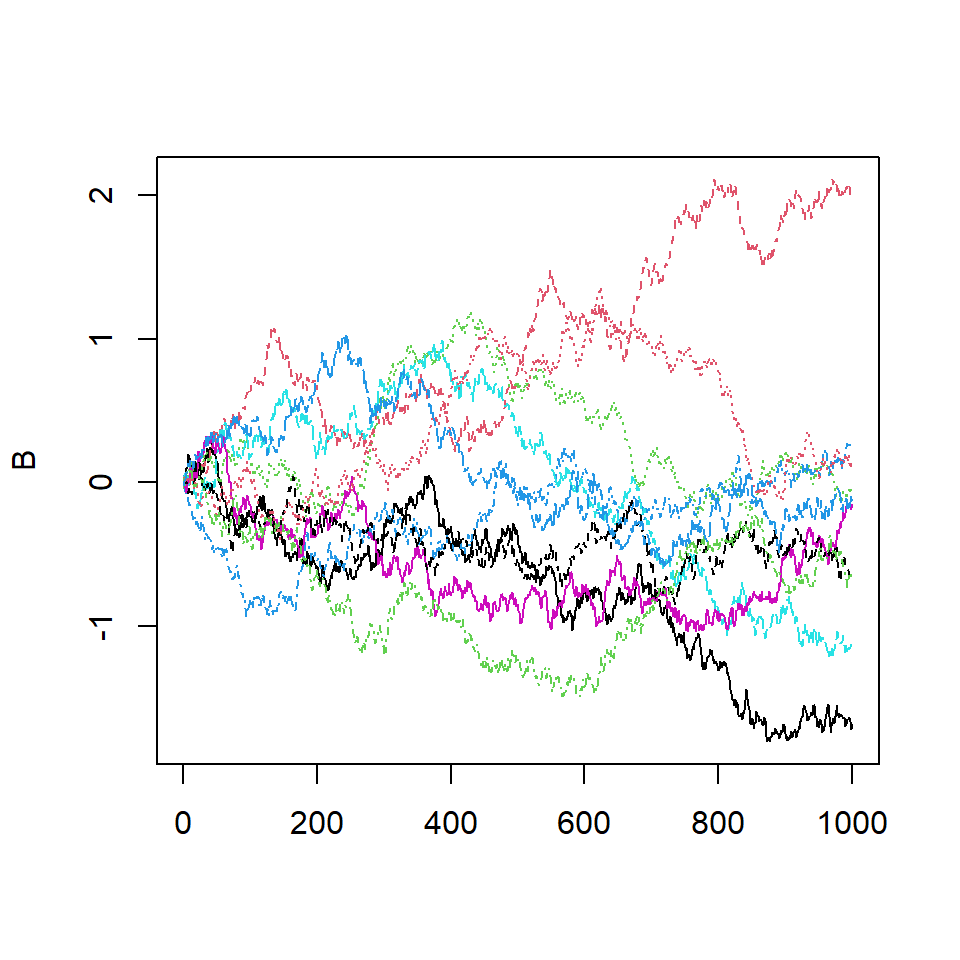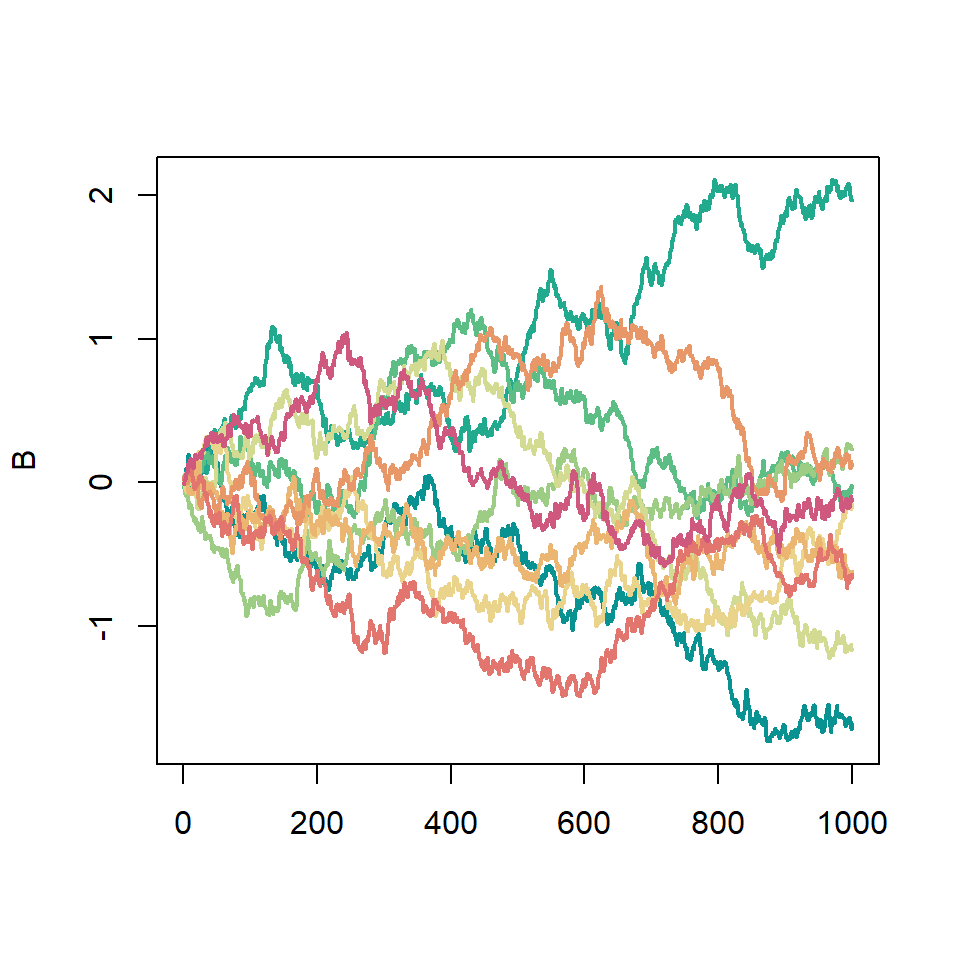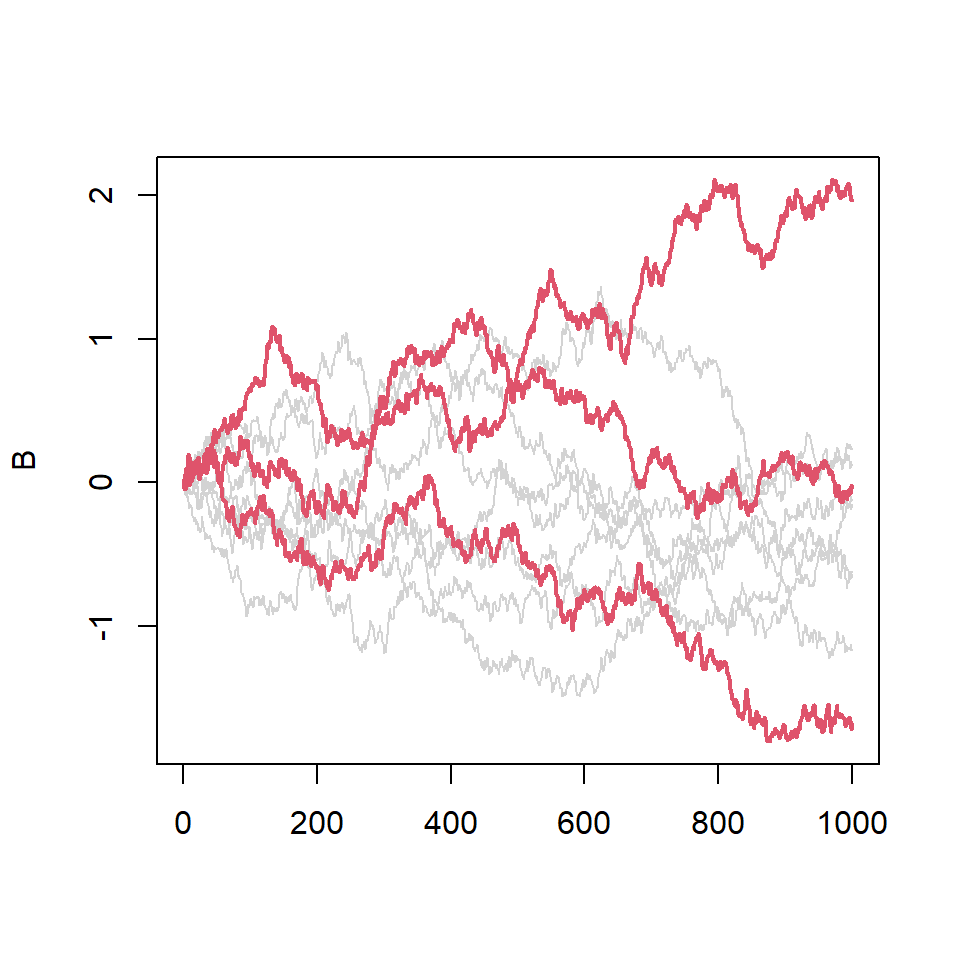# Plotting columns in R with matplot and matlines functions

## Sample data set

For this tutorial we are going to use the following sample data, which consists on ten realizations of a brownian motion. The corresponding data for each path is a column of the `B` matrix.

``````# Brownian motion
set.seed(1)

# Grid
t <- seq(0, 1, by = 0.001)
p <- length(t) - 1

# 10 paths
n <- 10
I <- matrix(rnorm(n * p, 0, 1 / sqrt(p)), n, p)

# Matrix
B <- apply(I, 1, cumsum)``````

## `matplot` function

In order to create a line chart with all the columns of the data at the same time you can make use of `matplot` and setting `type = "l"`. Note that `B` is a numeric matrix, but could also be a data frame or a vector and a matrix.

``````matplot(B, type = "l")

# Equivalent to:
matplot(as.data.frame(B), type = "l")

# Equivalent to:
matplot(matrix(t), rbind(rep(0, n), B), type = "l")``````By default, the function uses colors 1 to 6, line width of 1 and line types 1 to 5 to create the lines, but his can be overridden with arguments `col`, `lwd` and `lty`, respectively.

``````# Colors
cols <- hcl.colors(10, "Temps")

matplot(B, type = "l",
col = cols, # Colors
lwd = 2,    # Line width
lty = 1)    # Line type``````## `matlines` functionThe `matlines` function is to `matplot` the same as `lines` is to `plot`. It allows you adding more lines from a data frame or matrix to the previous plot. In the following example we are using it to highlight some paths of the brownian motion.

``````matplot(B, type = "l",
col = "lightgray",
lty = 1)

# Highlight the first three columns
# with a different color
matlines(B[, 1:3], type = "l",
col = 2, lwd = 2,
lty = 1)``````